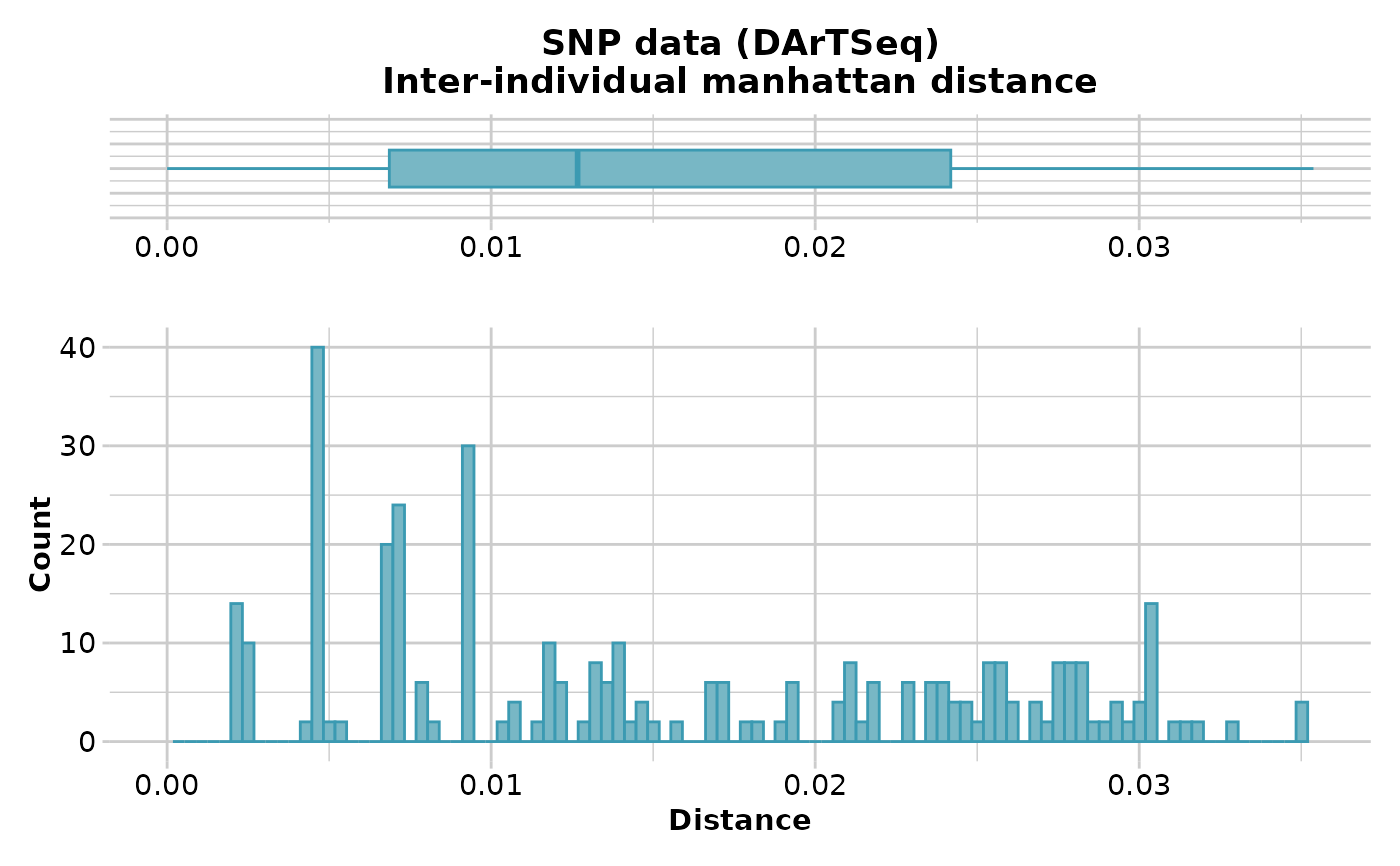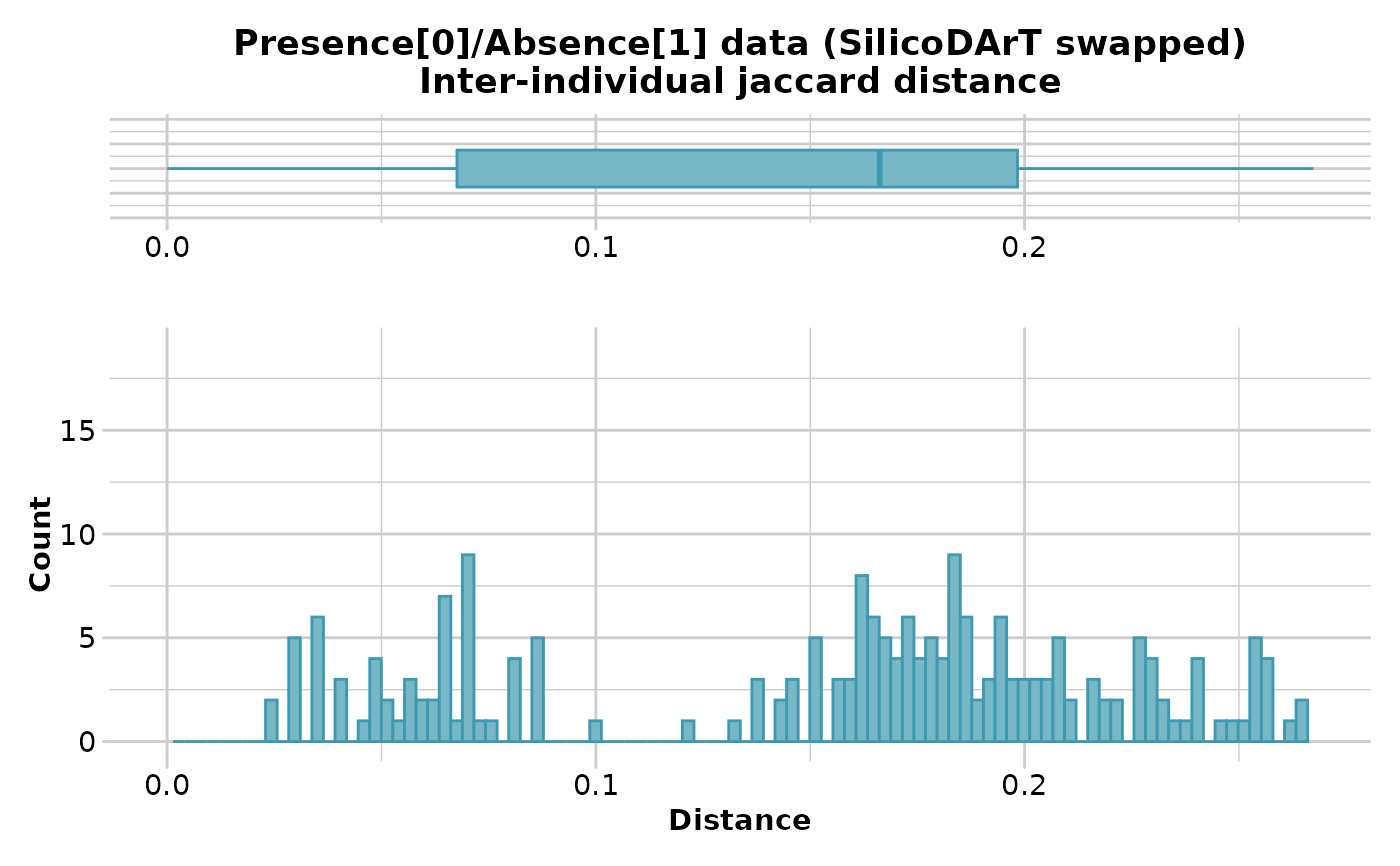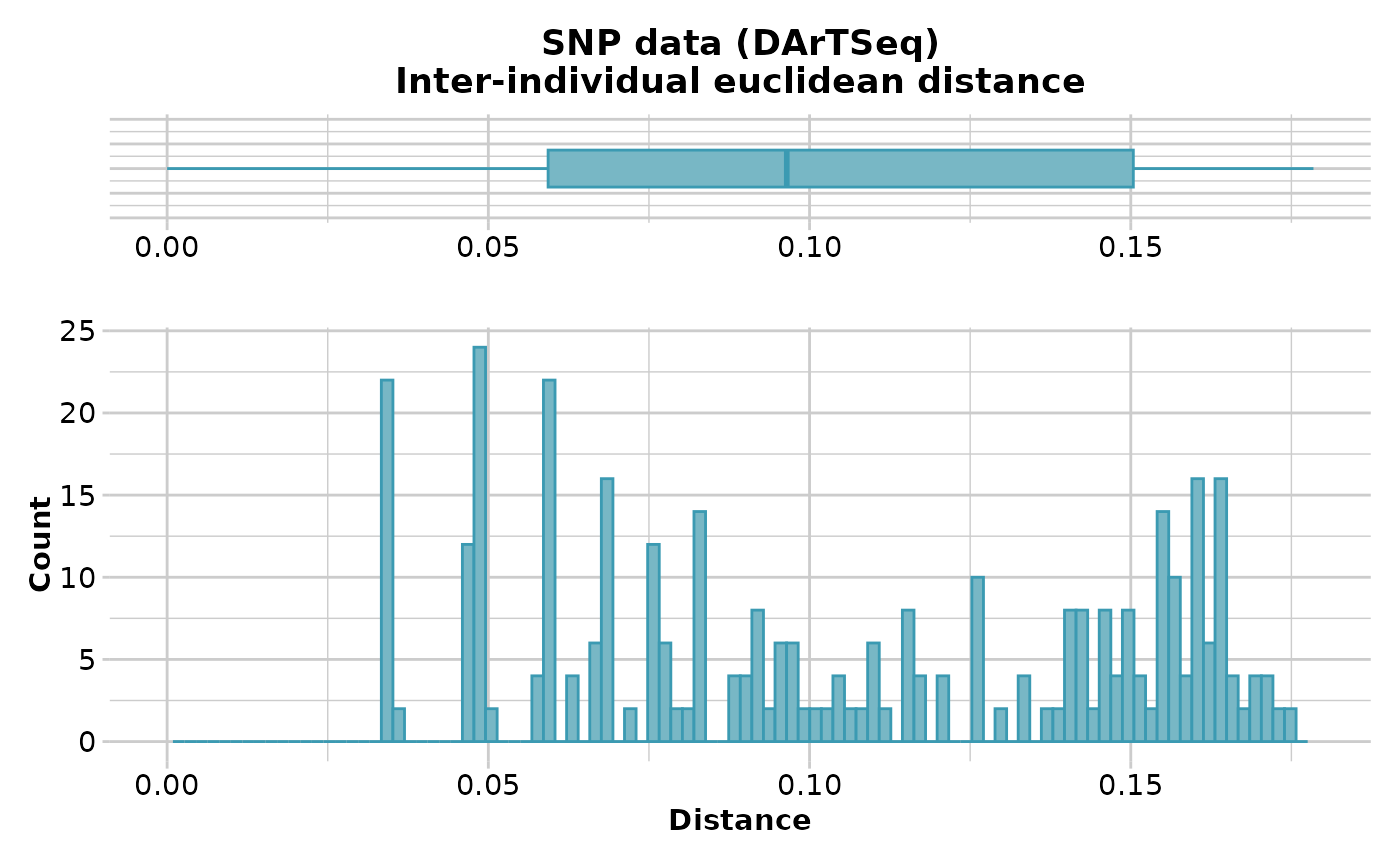This script calculates various distances between individuals based on allele frequencies or presence-absence data

## Usage

gl.dist.ind(
x,
method = NULL,
scale = FALSE,
swap = FALSE,
output = "dist",
plot.out = TRUE,
plot_theme = theme_dartR(),
plot_colors = two_colors,
save2tmp = FALSE,
verbose = NULL
)

## Arguments

x

Name of the genlight containing the SNP genotypes or presence-absence data [required].

method

Specify distance measure [SNP: Euclidean; P/A: Simple].

scale

If TRUE, the distances are scaled to fall in the range [0,1] [default TRUE]

swap

If TRUE and working with presence-absence data, then presence (no disrupting mutation) is scored as 0 and absence (presence of a disrupting mutation) is scored as 1 [default FALSE].

output

Specify the format and class of the object to be returned, 'dist' for a object of class dist, 'matrix' for an object of class matrix [default "dist"].

plot.out

If TRUE, display a histogram and a boxplot of the genetic distances [TRUE].

plot_theme

User specified theme [default theme_dartR].

plot_colors

Vector with two color names for the borders and fill [default two_colors].

save2tmp

If TRUE, saves any ggplots to the session temporary directory [default FALSE].

verbose

Verbosity: 0, silent or fatal errors; 1, begin and end; 2, progress log ; 3, progress and results summary; 5, full report [default 2 or as specified using gl.set.verbosity].

## Value

An object of class 'matrix' or dist' giving distances between individuals

## Details

The distance measure for SNP genotypes can be one of:

• Euclidean Distance [method = "Euclidean"]

• Scaled Euclidean Distance [method='Euclidean", scale=TRUE]

• Simple Mismatch Distance [method="Simple"]

• Absolute Mismatch Distance [method="Absolute"]

• Czekanowski (Manhattan) Distance [method="Manhattan"]

The distance measure for Sequence Tag Presence/Absence data (binary) can be one of:

• Euclidean Distance [method = "Euclidean"]

• Scaled Euclidean Distance [method='Euclidean", scale=TRUE]

• Simple Matching Distance [method="Simple"]

• Jaccard Distance [method="Jaccard"]

• Bray-Curtis Distance [method="Bray-Curtis"]

Refer to the dartR Technical Note on Distances in Genetics.

## Author

Author(s): Arthur Georges. Custodian: Arthur Georges -- Post to #' https://groups.google.com/d/forum/dartr

## Examples

 # \donttest{
D <- gl.dist.ind(testset.gl[1:20,], method='manhattan')
#> Starting gl.dist.ind
#>   Processing genlight object with SNP data
#>   Warning: data include loci that are scored NA across all individuals.
#>   Consider filtering using gl <- gl.filter.allna(gl)
#>   Calculating Manhattan distance
#>#>   Returning a stat::dist object
#> Completed: gl.dist.ind
#>
D <- gl.dist.ind(testset.gs[1:20,], method='Jaccard',swap=TRUE)
#> Starting gl.dist.ind
#>   Processing genlight object with Presence/Absence (SilicoDArT) data
#>   Calculating distances based on the Jaccard Coefficient
#>#>   Returning a stat::dist object
#> Completed: gl.dist.ind
#>
# }
D <- gl.dist.ind(testset.gl[1:20,], method='euclidean',scale=TRUE)
#> Starting gl.dist.ind
#>   Processing genlight object with SNP data
#>   Warning: data include loci that are scored NA across all individuals.
#>   Consider filtering using gl <- gl.filter.allna(gl)
#>   Calculating scaled Euclidean Distances between individuals
#>#>   Returning a stat::dist object
#> Completed: gl.dist.ind
#>